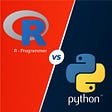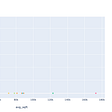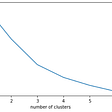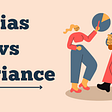# Random Effects Model

Linear regression is a mathematical approach to model the relationship between a scalar dependent variable (also called response variable) and one or more than one independent variables (also called explanatory variable). It is a linear model, assuming a linear relationship between dependent and independent variables.

There are two kinds of linear regression models based on the type of relationship between variables.

1. Fixed effects model
2. Random effects model

There is a third model, called the mixed effects model. It is basically a combination of fixed and random effect model.

# Fixed effects model

This is a category of the model where the response (dependent variable) is the only variable that changes with respect to different levels of independent variable, i.e. the linear relationship remains same for all levels. This is the most fundamental linear regression model.

The most common examples of fixed effects model are: categorical variable for gender or race.

# Random effects model

Unlike a fixed effect model, the relationship between dependent variable and independent variable changes with different levels of the independent variable. In other words: “A random-effect assumes that the explanatory variables have a fixed relationship with response variables across all observations, but that these fixed effects may vary from one observation to another”.

The most common examples of mixed effects model is when we have list of countries as an independent variable. In this example, we can use random effects for a country which will helps us incorporate the variability which can occur because of data being present from some specific country or due to varying internal situations.

# Fixed-effect vs Random-effect model

Another fundamental difference between fixed and random effect model is that fixed effect model allows prediction only about the level / categories of the dependent variable present in the training data, whereas a random-effect model allows us to make inference about the population, i.e. make predictions about level / categories not present in the training data.

# References

1. Jason Brownlee, Linear Regression for Machine Learning, 2016, Machine Learning Mystery.
2. George Farkas, Fixed-effects model, 2005, ScienceDirect.
3. Ajithesh Kumar, Fixed vs Random vs Mixed Effects Models -Examples, 2021, Data Analytics.
4. Neil Salkind, Fixed-effects models, 2010, researchmethods.

--

--

--

## More from Harsh

Data Science Enthusiast

Love podcasts or audiobooks? Learn on the go with our new app.

## Experience Analysis through Weather Data : Exploratory Data Analysis## Understanding the ETL process and considering best practices## Designing the 15 Minute City with AirBnB Reviews and Semantic AI## R Programming Vs Python Programming## Donuts & Coffee Meet The City Economy## Communicating with Data## Harsh

Data Science Enthusiast

## Bagging vs Boosting in Machine Learning training process## Feature Selection With Lasso Regression## The intuition behind K-means clustering technique## What are Bias and Variance? Difference and relation between Bias and Variance: